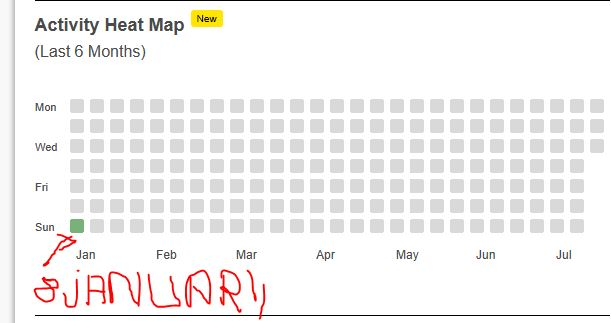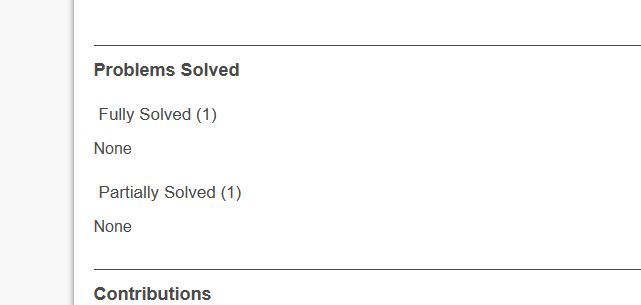# Do zco and inoi real time olympiad allow us submit problems multiple times?

Do zco and inoi real time olympiad allow us submit problems multiple times?
if yes then how many times.

Today i came across one participant zco id profile , he had only submitted on jan 8 when olympiad was held with 2 problem of 3 hrs duration .The total number of problem was 2 , so how his solution get partially accepted 7 times, wrong answer 1 time , runtikme error 1 time , compile error 1 time and full solution accepted 1 time

**And overall his submission was 1 fully accepted and 1 partially accepted out of 2 problem **His profile link:–> cextzco9424420 | CodeChef User Profile for ZCO 2023 user 42442 | CodeChef

-=-=-=-=-=-=-=-=-=-=-=-=-=-=-=-=-=-=-=-=-=-=-=-=-=-=-=-=-=-=-=-=-=-=-=-=-=-=-=-=-=-=-=-=-=-=-=-=-=-=-=-=-=-=-=-=-=-=-=-=-=-=-=-=-=-=-=-=-=-=-=-=-=-=-=-=-=-=-=-=-=-=-=-=-=-=-=-=-=-=-=-=-=-==-=-=-=-==-=-=-=-=-==-==-==-=-=-==-=-=-=-=-==-=-=-=-=-==–==–==-=-=-=-=-==-=-=-==-=-=-=-=-==-=-==-=-=-==-=-=-=-=-=-=-=-==-==-=-=–==-=-=-==-=-=-===-=-=-=-==-=-=-=-=-=-=-=-=-=-=

@k_k_1729 i thinks it’s your profile as you have got liked by @cextzco9424420